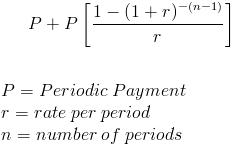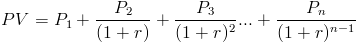# Present Value of Annuity Due

PV of Annuity Due Calculator (Click Here or Scroll Down)The formula for the present value of an annuity due, sometimes referred to as an immediate annuity, is used to calculate a series of periodic payments, or cash flows, that start immediately.

## How is the Present Value of an Annuity Due Derived?

The present value of an annuity due formula uses the same formula as an ordinary annuity, except that the immediate cash flow is added to the present value of the future periodic cash flows remaining. The number of future periodic cash flows remaining is equal to n - 1, as n includes the first cash flow.

To show this visually, the extended version of the present value of annuity due formula ofshows that the first cash flow is not discounted and that the discounted cash flows start at period 2. After factoring out the first immediate payment, the additional payments consist of an ordinary annuity with n - 1 payments remaining.

The formula shown on the top of the page can be shown as P + PV of ordinary
annuityn-1.

## Alternative Formula for the Present Value of an Annuity Due

The present value of an annuity due formula can also be stated aswhich is (1+r) times the present value of an ordinary annuity. This can be shown by looking again at the extended version of the present value of an annuity due formula ofThis formula shows that if the present value of an annuity due is divided by (1+r), the result would be the extended version of the present value of an ordinary annuity ofIf dividing an annuity due by (1+r) equals the present value of an ordinary annuity, then multiplying the present value of an ordinary annuity by (1+r) will result in the alternative formula shown for the present value of an annuity due.

New to Finance?